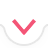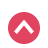# Word Problems

Quiz History

Select Topics
Age

Distance

Mixture

Work

Investment
Start Quiz
Quiz Assignment

00:00:00Summary
Previous Question

Show Hints

3 / 3
Sorry, your browser does not support this application
x^2
x^3
x^4
x^{\msquare}
\frac{\msquare}{\msquare}
(\square)
\sqrt{\square}
\nthroot{\square}
\mathrm{remainder}
+
-
\cdot
Let's Try Again :(
Try to simplify further
Thank you!
Start Quiz 00:00:00
Previous Question
1 / 10
x^2
x^3
x^4
x^{\msquare}
\frac{\msquare}{\msquare}
(\square)
\sqrt{\square}
\nthroot{\square}
\mathrm{remainder}
+
-
\cdot
Finish Quiz

Symbolab

# Summary

Submit Assignment Start Over Back

# Summary

Start Again Back
Prev Student Next Student

in 01:12:13 hoursBack
Back

## Steps

Sorry, your browser does not support this application
Edit Quiz
Quiz Saved!# Crowns

You haven't collected any crowns yet

# Quizzes